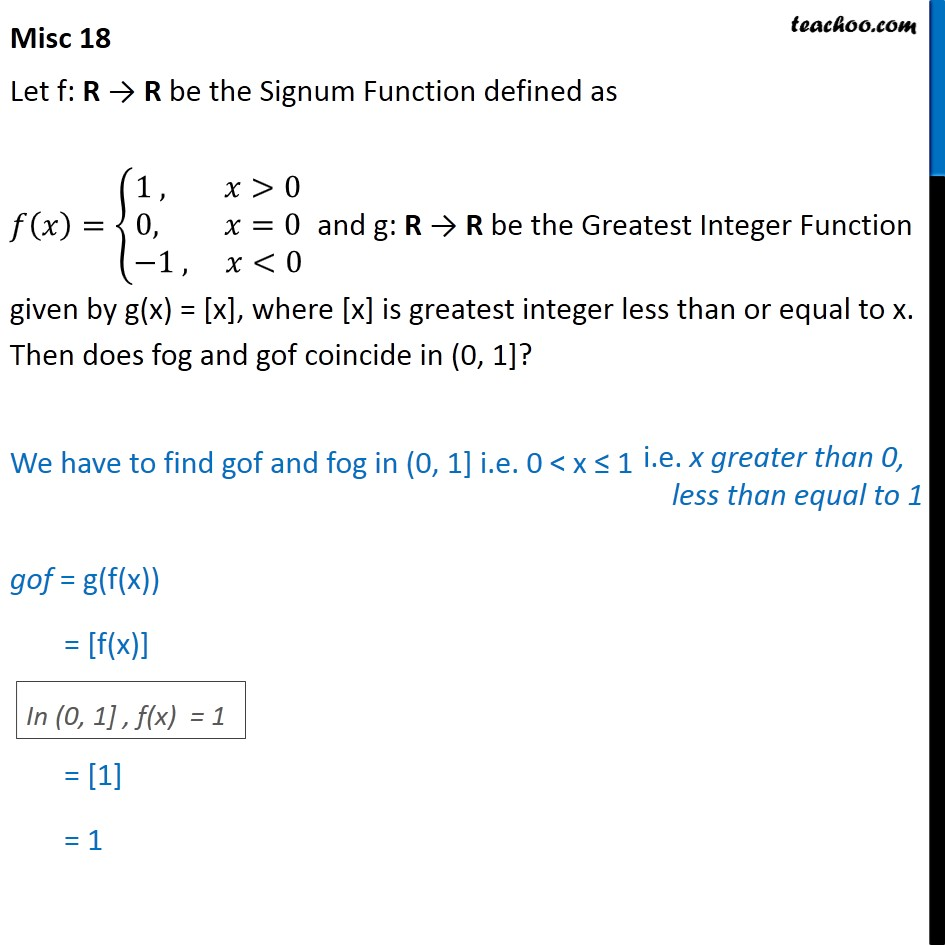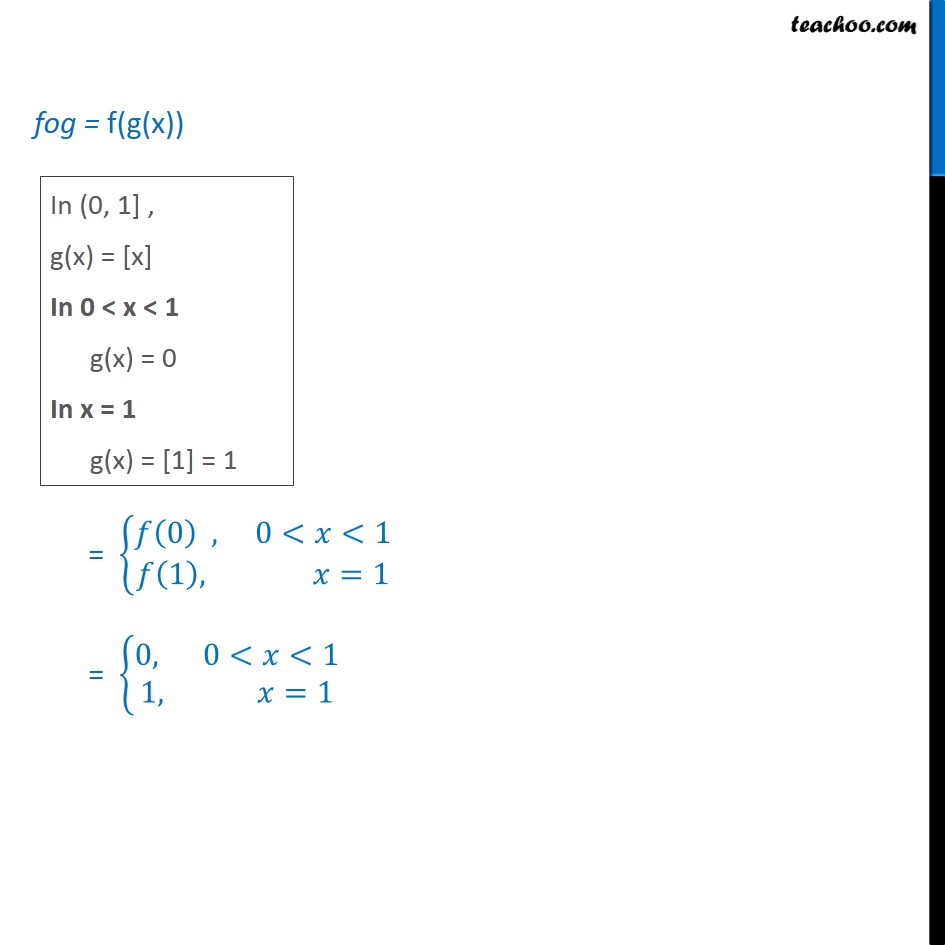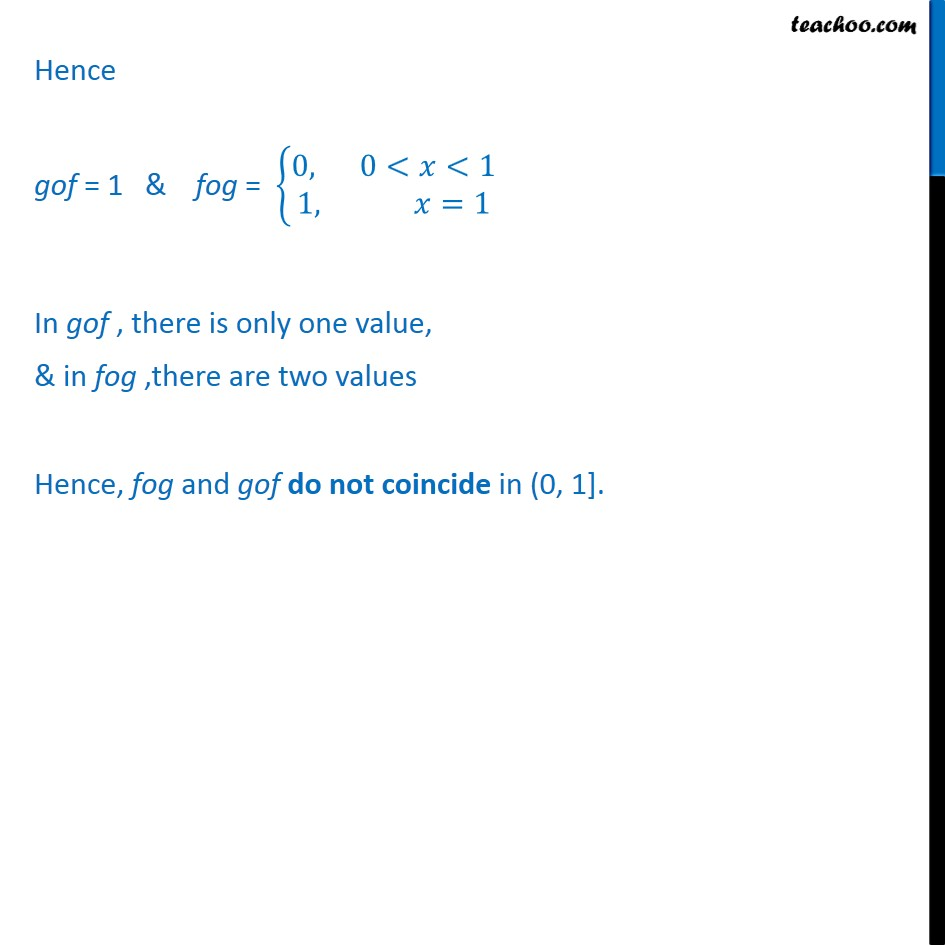Composite functions

Chapter 1 Class 12 Relation and Functions
Concept wiseIntroducing your new favourite teacher - Teachoo Black, at only ₹83 per month

### Transcript

Misc 18 Let f: R → R be the Signum Function defined as 𝑓 𝑥﷯= 1 , 𝑥>0﷮&0, 𝑥=0﷮−1 , 𝑥<0﷯﷯ and g: R → R be the Greatest Integer Function given by g(x) = [x], where [x] is greatest integer less than or equal to x. Then does fog and gof coincide in (0, 1]? We have to find gof and fog in (0, 1] i.e. 0 < x ≤ 1 gof = g(f(x)) = [f(x)] =  = 1 fog = f(g(x)) = 𝑓 0﷯ , 0<𝑥<1﷮𝑓 1﷯, 𝑥=1﷯﷯ = 0, 0<𝑥<1﷮1, 𝑥=1﷯﷯ Hence gof = 1 & fog = 0, 0<𝑥<1﷮1, 𝑥=1﷯﷯ In gof , there is only one value, & in fog ,there are two values Hence, fog and gof do not coincide in (0, 1].